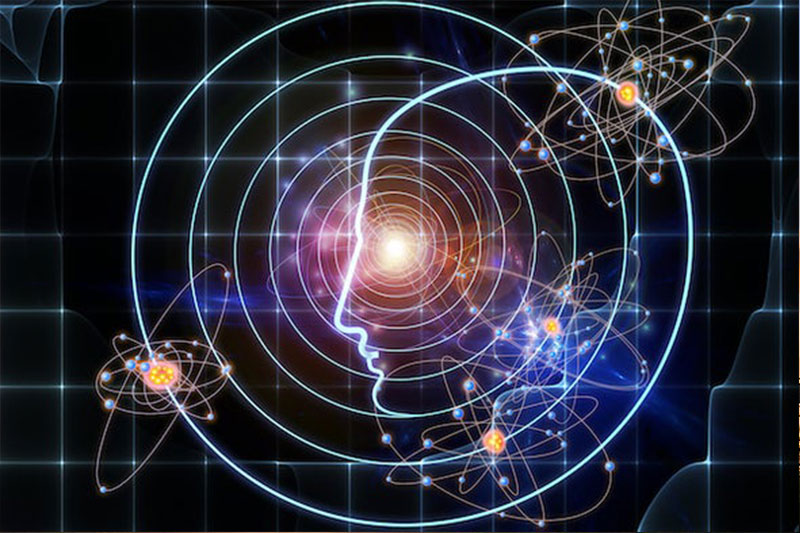Uncertainty Principle of Metaphysical World! | Akhand Gyan | Eternal Wisdom

# Uncertainty Principle of Metaphysical World!In quantum mechanics, the famous Uncertainty Principle describes certain mathematical inequalities, emphasizing a fundamental limit to the accuracy with which some pairs of physical properties of a particle can be found. These pairs are known as complementary variables or canonically conjugate variables. For example: position 'x' and momentum 'p', energy 'E' and time 't'.

The uncertainty Principle or Indeterminacy Principle, also called Heisenberg Uncertainty Principle, was first articulated in 1927 by the German physicist Werner Heisenberg. This renowned principle states that the position (x) and the momentum (p) of a particle cannot be measured simultaneously with absolute precision. In other words, the more accurately we measure one physical property, the other becomes highly uncertain. If the position of some particle is determined precisely, the accuracy in its momentum falls drastically (or, we can say, the error in finding its momentum shoots up significantly), and vice versa.

Mathematically, Uncertainty Principle  is expressed as:

∆X∆p ≥ h/2              (1)

Where,
∆X = uncertainty in the position

∆p = uncertainty in the momentum

h =reduced Planck constant, h/(2π)-

∆E∆t ≥ h/2              (2)

Where
∆E = uncertainty in the energy

∆t = uncertainty in the time

That is, the product of the uncertainties or the errors in the measurements (represented by the triangle symbol (∆), the Greek letter 'delta' before each variable) of these complementary variables is equal to or greater than half of a constant called "h-bar", which is equivalent to Planck's constant (written as h) divided by 2x. We cannot measure concurrently both the complimentary variable with perfect accuracy.

Equation (1) depicts the uncertainty relation between position and momentum.

Equation (2) depicts the uncertainty relation between energy and time.

But how this vital conclusion of quantum mechanics has one to one correlation with the metaphysical world? To know this and furthermore, read the complete article in Sept'19 edition of English Akhand Gyan Monthly Magazine.